# Solutions for Practice session 1¶

### Exercise 1. Main concepts in classification.¶

Consider the task of deciding whether a pet is a cat or a dog, given its weight and height. To learn a classifier, we are given data about 5 cats and 5 dogs. The weights of the 5 cats are $4, 5, 5, 5, 6$ units and the heights are $2, 1, 2, 3, 2$ units. The weights of the 5 dogs are $7, 11, 11, 13, 13$ units and the heights are $4, 8, 10, 8, 10$ units. For convenience, let us call the weight units as pettygrams and the height units as pettymeters.

(a) How many instances are there? How many training instances? How many test instances? How many features? How many classes?

Answer: There are 10 instances - 5 dogs and 5 cats. We will treat them all as training instances in our solution, learning the classifier making use of all these 10 instances. Thus, there are no test instances. In principle, it is also possible to use less instances for training, keeping the rest for testing. There are 2 features - height and weight. There are 2 classes - $\mathsf{cat}$ and $\mathsf{dog}$.

(b) What is the input space $\mathcal{X}$? What is the output space $\mathcal{Y}$?

Answer: The input space is the space of all possible feature vectors of instances. As there are two numeric features, then $\mathcal{X}$ is the set of all pairs of real numbers, $\mathcal{X}=\mathbb{R}\times\mathbb{R}=\mathbb{R}^2$. Since weight and height are all positive numbers, then we could define instead $\mathcal{X}=(0,\infty)\times(0,\infty)$. The output space is the space of all possible labels, $\mathcal{Y}=\{\mathsf{cat},\mathsf{dog}\}$. For mathematical convenience, it is often useful to call one class as positive and denote by $1$ (sometimes positivity is emphasised in notation as $+1$) and another class as negative and denote by $-1$. With such notation, $\mathcal{Y}=\{-1,+1\}$.

(c) What is the first instance $\mathbf{x}_1$ here? Write it down in mathematical vector notation, that is $(\dots)$. What is its label $y_1$?

Answer: The order of instances has not been given very explicitly and for often it does not matter much either. As cats were mentioned earlier, we could have the first cat as $\mathbf{x}_1$, that is $\mathbf{x}_1=(4,2)$, meaning that the value of the first feature (weight) is $4$ (pettygrams) and the value of the second feature (height) is $2$ (pettymeters).

(d) Choose one of the classes to be called as positives and the other as negatives. Write down the contents of $\mathsf{Tr}^{\bigoplus}$ in mathematical set and vector notation, that is: $\{(\dots),(\dots),\dots\}$.

Answer: Let's choose the class $\mathsf{cat}$ to be the negative class and $\mathsf{dog}$ to be the positive class (an arbitrary choice, but perhaps it is more intuitive to have instances with higher feature values to be positive). Then $\mathsf{Tr}^{\bigoplus}=\{(7,4),(11,8),(11,10),(13,8),(13,10)\}$.

(e) Present the data as a single table.

Answer: Let us start using the numpy and pandas packages in Python.

In :
import pandas as pd
import numpy as np

In :
data = pd.DataFrame({
'weight': [4, 5, 5, 5, 6, 7, 11, 11, 13, 13], # in pettygrams
'height': [2, 1, 2, 3, 2, 4, 8,  10, 8,  10], # in pettymeters
'label':  ['cat']*5 + ['dog']*5
},
columns = ['weight', 'height', 'label'] # mantains column order
)
data

Out:
weight height label
0 4 2 cat
1 5 1 cat
2 5 2 cat
3 5 3 cat
4 6 2 cat
5 7 4 dog
6 11 8 dog
7 11 10 dog
8 13 8 dog
9 13 10 dog

Here we have chosen to have instances as rows and attributes (features and label) as columns. While in principle it could be the other way around, the chosen way of presenting the data is standard in machine learning.

(f) Visualise the data. Are the classes linearly separable? Can we use only one feature (weight or height) to separate the data?

Answer: For visualisation, we will use the matplotlib package first, and later show an alternative with the seaborn package.

In :
from matplotlib import pyplot as plt


Minimal code to get some first visualisation:

In :
plt.plot(data['weight'],data['height'],'o')
plt.show()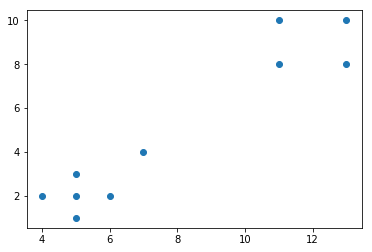Let us now use red for dogs (positive class) and blue for cats (negative class).

In :
def plot_cats_and_dogs(data):
plt.plot(data.loc[data.label=='cat', 'weight'], data.loc[data.label=='cat', 'height'], 'bo', label='cats')
plt.plot(data.loc[data.label=='dog', 'weight'], data.loc[data.label=='dog', 'height'], 'ro', label='dogs')

plt.legend(markerscale=1)

# Specify axes parameters
plt.xticks(list(range(0, 15)))
plt.yticks(list(range(1, 12)))
plt.xlim(0, 14)
plt.ylim(0, 11)

# Axis labels
plt.xlabel('Weight')
plt.ylabel('Height')

plot_cats_and_dogs(data)
plt.show()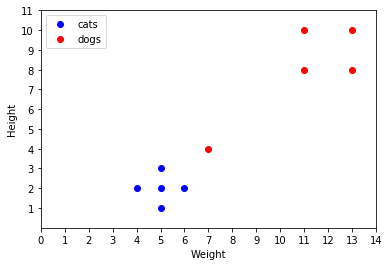An alternative package for plotting is seaborn:

In :
import seaborn as sns

In :
sns.lmplot(data=data,     # set datafrafe
x='weight',    # set column used for x
y='height',    # and y
hue='label',   # specify color
fit_reg=False  # don't fit any regression
)
plt.show()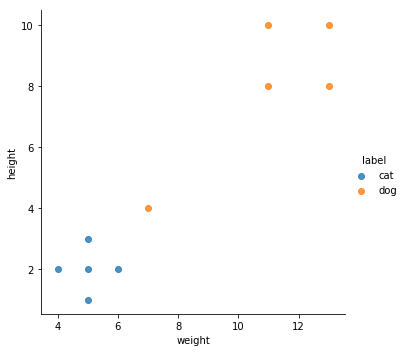Yes, the classes are linearly separable, as we can easily draw a straight line such that all dogs are on one side and all cats are on the other side. We can also use any one of the features to linearly separate the data, e.g. all dogs have $\mathsf{weight}\geq 7$ and all cats have $\mathsf{weight}<7$.

### Exercise 2. Basic linear classifier.¶

(a) Calculate and visualise the class centres $\mathbf{p}$ and $\mathbf{n}$.

Answer: In the code we will refer to $\mathbf{p}$ and $\mathbf{n}$ as average_dog and average_cat.

In :
average_cat = np.array((np.mean(data.loc[data.label == 'cat', 'weight']), np.mean(data.loc[data.label == 'cat', 'height'])))
average_dog = np.array((np.mean(data.loc[data.label == 'dog', 'weight']), np.mean(data.loc[data.label == 'dog', 'height'])))

# Re-use our plot
plot_cats_and_dogs(data)

plt.plot(*average_cat, 'b+', markersize=15, label='average cat')
plt.plot(*average_dog, 'r+', markersize=15, label='average dog')

plt.legend()
plt.show()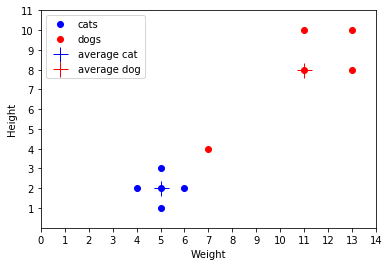(b) For any instance $\mathbf{x}$, the basic linear classifier predicts positive or negative, depending on whether $\mathbf{x}$ is closer to $\mathbf{p}$ or to $\mathbf{n}$. Does it predict the correct class on all training instances?

Answer: No, there is one dog with $\mathsf{weight}=7$ and $\mathsf{height=4}$ which is closer to the average cat $\mathbf{n}=(5,2)$ than to the average dog $\mathbf{p}=(11,8)$. This dog will be predicted as cat by the basic linear classifier.

(c) Calculating distances requires taking squares and square roots, in what sense is this then a linear classifier?

Answer: The decision boundary (all points which are at equal distance from the average cat and average dog) is straight line, and therefore, the classifier can be represented by thresholding a linear function of feature values.

(d) Find vector $\mathbf{w}$ and real number $t$, such that the basic linear classifier predicts positive if $\mathbf{w}\cdot\mathbf{x}>t$ and negative if $\mathbf{w}\cdot\mathbf{x}<t$. Apply this to demonstrate that one training instance is misclassified.

Answer: We will use the formulas $\mathbf{w}=\mathbf{p}-\mathbf{n}$ and $t=(\mathbf{p}-\mathbf{n})\cdot(\mathbf{p}+\mathbf{n})/2$ from the lecture slides.

In :
w = average_dog - average_cat
t = np.dot(average_dog - average_cat, average_dog + average_cat) / 2
print(w)
print(t)

[ 6.  6.]
78.0


We will now show that the basic linear classifier classify the instance $(7,4)$ as negative, while the actual label is positive (dog).

In :
predicted_class = np.sign( np.dot(w,np.array((7,4))) - t )
predicted_class

Out:
-1.0

(e) What should the basic linear classifier predict for instances that are exactly on the decision boundary, that is $\mathbf{w}\cdot\mathbf{x}=t$? Draw the decision boundary.

Answer: This depends on the exact definition of the basic linear classifier. The definition given in the lecture implies positive prediction for $\mathbf{w}\cdot\mathbf{x}>t$ and negative otherwise, therefore at the border it predicts negative. However, this is arbitrary, and the definition could as well be changed to predict positive at the border. The definition could even state that at the border the model would predict the class randomly, with equal probability $1/2$ for both classes. In practice, the behaviour exactly at the border almost never matters, because it is unlikely that any instance would be exactly at the border. We will draw the decision boundary by calculating where it crosses the axes. The crossing point with the y-axis can be obtained by setting $\mathsf{weight}=0$ and thus $\mathbf{w}\cdot(0,\mathsf{height})=t$ and hence $\mathsf{height}=t/w_2$. Similarly, the crossing point with the x-axis is $\mathsf{weight}=t/w_1$.

In :
crossing_y = t/w # since indexing in Python starts from 0
crossing_x = t/w
#plt.plot(*list(zip(avg_cat, avg_pt)), 'b--')
#plt.plot(*list(zip(avg_pt, avg_dog)), 'r--')
plot_cats_and_dogs(data)
plt.plot([0, crossing_y], [crossing_y, 0], 'k--')

plt.legend()
plt.show()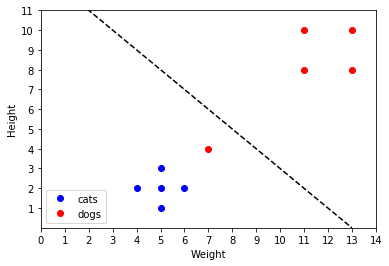(f) Does there exist a linear classifier which predicts correctly on all the training instances? Try to find one such classifier by changing $t$. If you can find one, then draw the new decision boundary.

Answer: Visually it is easy to notice that the current decision boundary can be shifted downwards such that it would go below all dogs and above all cats. To find the suitable threshold $t$, we calculate $\mathbf{w}\cdot\mathbf{x}$ for the misclassified dog and for the highest cat, and set $t$ to be between these values.

In :
t_dog = np.dot(w,(7,4))
t_cat = np.dot(w,(5,3))
t_new = (t_dog+t_cat)/2
print('New threshold t is:',t_new)

new_crossing_y = t_new/w
new_crossing_x = t_new/w
plot_cats_and_dogs(data)
plt.plot([0, new_crossing_y], [new_crossing_y, 0], 'k--')

plt.legend()
plt.show()

New threshold t is: 57.0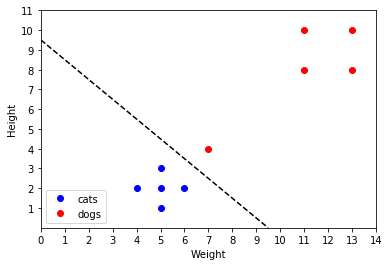### Exercise 3. Perceptron.¶

(a) Represent the basic linear classifier learned on the cats-and-dogs data in homogeneous coordinates.

Answer: All we need to do is to take $\mathbf{w}$ obtained above and assign the negated threshold $t$ as a bias term $w_0$, that is $w_0=-t$. Any instance $(\mathsf{weight},\mathsf{height})$ should now instead be represented with an additional coordinate with value $1$, i.e $(1,\mathsf{weight},\mathsf{height})$. We demonstrate this on the misclassified dog $(7,4)$.

In :
w_hom = np.array((-t,w,w))
x_hom = np.array((1,7,4))

predicted_class = np.sign( np.dot(w_hom,x_hom) )
predicted_class

Out:
-1.0

(b) Apply the first 30 iterations of the perceptron algorithm on the training data, that is 3 rounds (also called epochs) through the training instances. Initialise with the zero-vector and use the learning rate $\eta=1$. Did the algorithm converge yet? How many training instances are classified correctly by the model obtained after 30 iterations?

Answer: We start with $\mathbf{w}=(0,0,0)$.

Round 1. The first instance is $\mathbf{x}_1=(1,4,2)$ with label $y_1=-1$. As $y_1\mathbf{w}\cdot\mathbf{x}_1=0$, we go to change the weight vector as $\mathbf{w}\leftarrow\mathbf{w}+\eta y_1\mathbf{x}_1$, and hence $\mathbf{w}=(-1,-4,-2)$.

The second instance is $\mathbf{x}_2=(1,5,1)$ with label $y_2=-1$. As $y_2\mathbf{w}\cdot\mathbf{x}_2=23>0$, we continue to the next instance without changing the weights.

Similarly, there is no need to change the weights until we reach the first dog $\mathbf{x}_6=(1,7,4)$ and label $y_6=1$. As $y_6\mathbf{w}\cdot\mathbf{x}_6=-37$, we go to change the weight vector as $\mathbf{w}=(-1,-4,-2)+(1,7,4)=(0,3,2)$.

Again there is now no need to change the weights until we get back to the first cat $\mathbf{x}_1=(1,4,2)$ with label $y_1=-1$, that is to start Round 2. As $y_1\mathbf{w}\cdot\mathbf{x}_1=-16$, we go to change the weight vector as $\mathbf{w}=(0,3,2)-(1,4,2)=(-1,-1,0)$.

The next misclassified instance comes when we get to the first dog $\mathbf{x}_6=(1,7,4)$ and the weights become $\mathbf{w}=(-1,-1,0)+(1,7,4)=(0,6,4)$.

The next misclassified instance comes when we again get to the first cat $\mathbf{x}_6=(1,4,2)$, starting Round 3. The weights become $\mathbf{w}=(0,6,4)-(1,4,2)=(-1,2,2)$.

The second cat is $\mathbf{x}_2=(1,5,1)$ with label $y_2=-1$ and it gets also misclassified as $y_2\mathbf{w}\cdot\mathbf{x}_2=-11<0$. The weights become $\mathbf{w}=(-1,2,2)-(1,5,1)=(-2,-3,1)$.

The next misclassified instance comes when we get to the first dog $\mathbf{x}_6=(1,7,4)$ and the weights become $\mathbf{w}=(-2,-3,1)+(1,7,4)=(-1,4,5)$. The rest of the dogs are correctly classified and we reach the end of the 3rd epoch.

The model $\mathbf{w}=(-1,4,5)$ classifies all instances as positives (dogs) and thus is correct on 5 instances and wrong on 5 instances.

Note: if one would implement the algorithm then after 25 epochs the model converges into $\mathbf{w}=(-15,-5,13)$ which classifies all training instances correctly.

(c) What happens if we change the learning rate? What happens if we change the initial weights?

Answer: Changing the learning rate alone only changes the magnitude of $\mathbf{w}$ but not the convergence speed, because at every step the weights $\mathbf{w}$ are exactly $\eta$ times what they would be at the same step when running the algorithm with $\eta=1$. Once we change the initial weights, the results will also change, and $\eta$ also starts to make a difference.